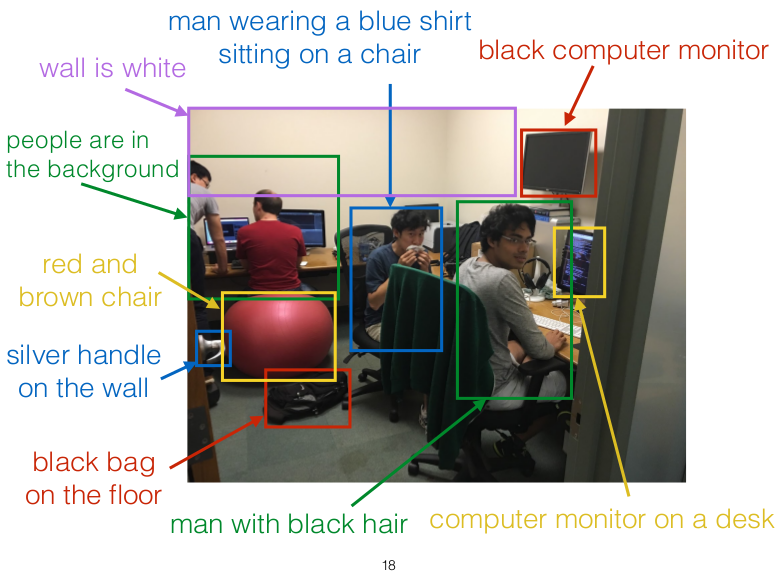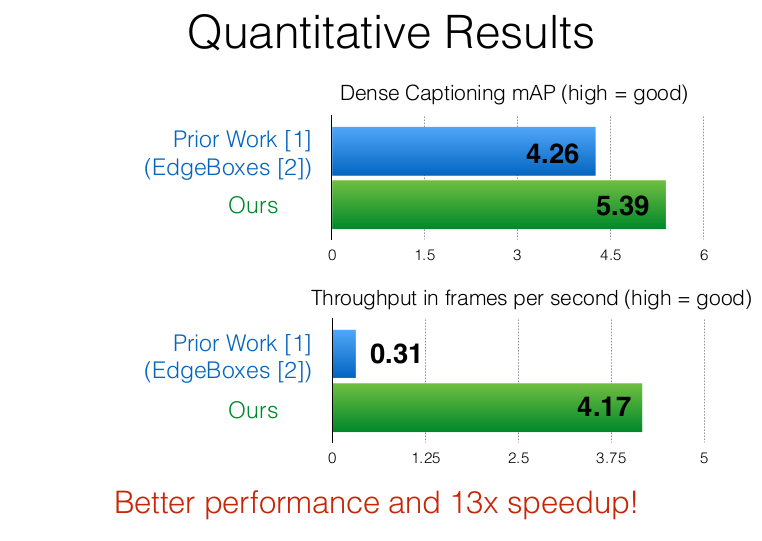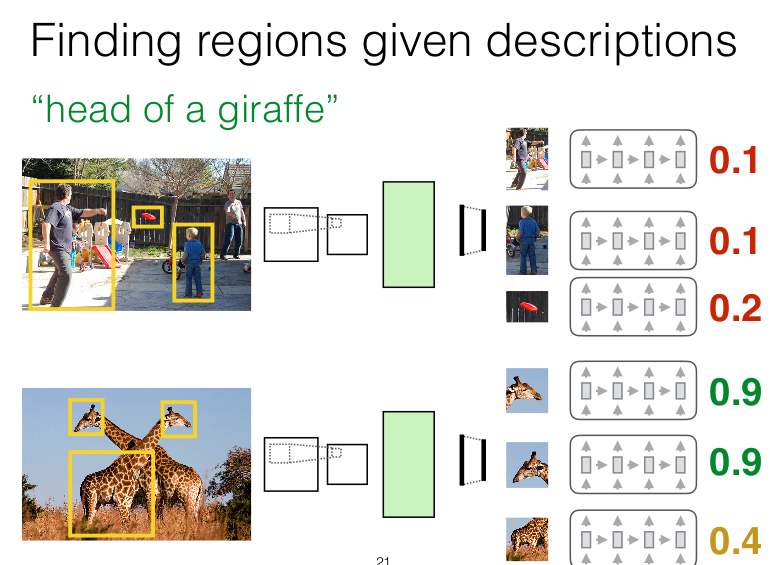PS：本篇博文不是对原文的简单翻译，论文中每一处涉及到的知识点以及论文中没有提及的技术细节，本文都会做一定的补充说明，如果还有什么看不懂的地方的话，可以留言一起讨论，我会尽量在24小时内回复。

(正文所有图片中的ksws0292756水印是我的CSDN博客)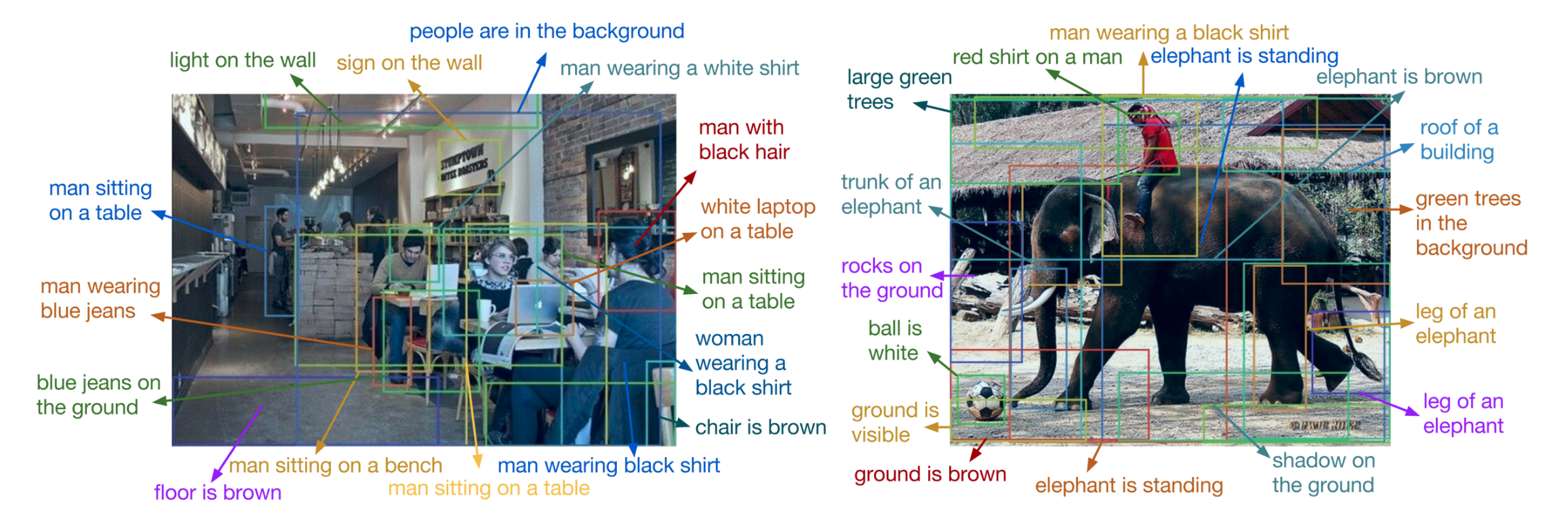# 摘要

这篇文章的主要工作是对图像的dense captioning。所谓dense captioning，就是要描述的对象不再是一幅简单的图片，而是要将图片中的许多局部细节都都用自然语言描述出来。这篇文章所做的工作可以说是object detection和image captioning的一般化，即当描述的语言是一个单词的时候，就可以看作是object detection，当描述的对象是整幅图片的时候，就成了普通的image captioning。

# 介绍

本小节主要介绍了dense cationing任务的定义，以及相对应的object detection和image caotioning方面的研究。大家可以自己看一下原文

# 相关工作

这里只给出重要的2篇论文（作者主要是在这两篇论文的几处上进行模型构建的），其他的可以参见原文

Faster R-CNN
http://papers.nips.cc/paper/5638-faster-r-cnn-towards-real-time-object-detection-with-region-proposal-networks
Deep Visual-Semantic Alignments for Generating Image Descriptions
https://cs.stanford.edu/people/karpathy/deepimagesent/

# 模型

## 模型框架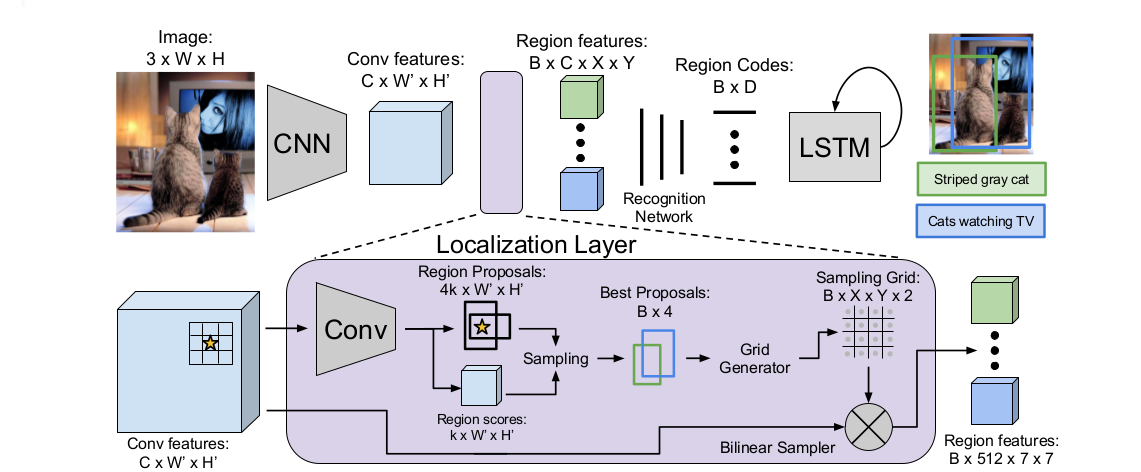### 卷积网络（Convalutional Network）

作者采用了基于VGG-16的网络结构，包含13层卷积核为3×3的卷积层和4层池化核为2×2的最大池化层（原本的VGG是5层池化层，这里作者做了一些小改动，改为4层），因此，对于大小为$3×W×H$的图片，经过卷积网络后，输出结果是$C×W’×H’$的特征图谱，这里$C=512$，$W’=\lfloor\frac{W}{16}\rfloor$，$H’=\lfloor\frac{H}{16}\rfloor$，该特征图谱就是下一层Fully Convolutional Localization Layer的输入。

### 全卷积定位层（Fully Convolutional Localization Layer）

: 来自卷积网络的特征图谱$C×W’×H’$（size任意）

: 输出B个候选区域的表征向量（定长），每个特征向量都包含下面三个关键信息：

• 候选区域的坐标：输出形式是一个$B×4$的矩阵，每行代表一个候选区域的坐标
• 候选区域的置信分数：一个长度为$B$的一维列向量，向量内每个元素都给出了候选区域的得分。得分越高说明越可能是真实区域
• 候选区域的特征：输出形式为$B×C×X×Y$的特征集合，这里B代表区域个数，$X×Y$表示特征图谱的大小（注意，这里的size已经是固定的），$C$代表特征的维度

这里额外说明一下，在CNN阶段作者不需要指定输入图片的大小（传统CNN分类任务由于FC全连接层的限制，使得输入图片的大小是固定的），因为这里作者关心的是图片的特征，而卷积层和池化层根本不care输出尺寸的多少，它们只负责拿到前一层的特征图谱（feature map）。
但是为什么这里的输出必须是定长的向量呢？主要是因为后面RNN模型的制约，由于RNN模型接受的数据必须的定长的，所以在全卷积定位层（FCL）阶段的最后一步，作者需要使用双线性插值的方法来使输出成为定长的特征向量。

这里的工作主要参考自Faster R-CNN。主要思想是借助一系列具有平移不变性的锚点（anchors）来预测候选区域的位置和大小，具体做法如下：
对于大小为$W’×H’$的特征图谱来说，将图谱中的每一个像素点都做为一个锚点（anchor）（锚点数量为$W’×H’$个），将该点反向映射会原始图像$W*H$中，然后基于该锚点，画出不同宽高比和大小的若干个“锚箱”（anchor box）。下图所示是3个具有相同大小但是不同宽高比的锚箱示例（分别为1:1，1:2，2:1）。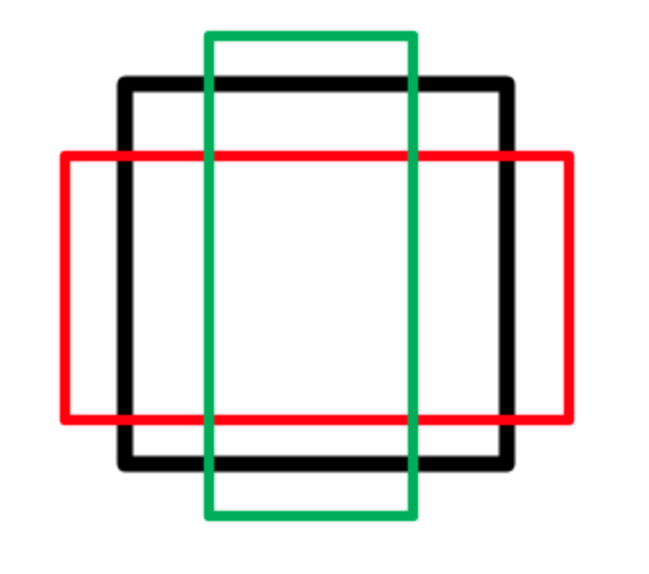如果采用Faster R-CNN的设置，即每个锚点对应3个不同的size取值（$128^2，256^2，512^2$）和3个不同的宽高比取值（1:1，1:2，2:1），因此，每个锚点对应的锚箱数量为$k=9$，在本文中采用的是$k=12$，具体对应多少个size和宽高比文中并没有给出。对于这$k$个锚箱，定位层（localization layer）会通过回归模型来预测相应的置信分数（score）和位置信息（scalars）。具体的计算过程是将特征图片作为输入，经过一个卷积核为$3×3$的卷积层（filter个数为256)，然后再经过一个卷积核为$1×1$卷积层（filter个数为$5k$，这里$k$代表anchor box的数量）,所以这一层的最终输出是$5k×W’×H’$的张量，包含了所有锚点对应的置信分数和位置信息。

边界回归主要是对刚刚预测的候选区域的一次精修，进行边界回归的原因主要是当前的候选区域可能与真实区域并不是特别匹配，如下图所示：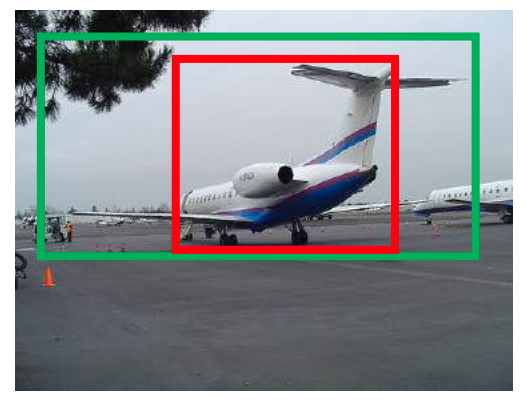图中，绿色框代表真实区域，红色框代表目前的候选区域，作者可以看到，候选区域虽然可以判断出区域内存在物体（飞机），但是它的定位并不是很准取，这时候就可以利用box regression来对边框进行微调。核心思想是利用线性回归得到关于边框的四个位移参数$（t_x,t_y,t_w,t_h）$，然后通过下面的式子对候选区域的中点$（x,y）$和size$（w，h）$进行更新

https://blog.csdn.net/zijin0802034/article/details/77685438
（PS：这篇论文的讲解是基于R-CNN的，其中的符号表示与本文有些出入，如$t_x,t_y$在R-CNN中代表的是真实区域的中心坐标，看的时候注意一下各个符号都表达了什么，不要搞混了）

以图像大小为$W=720，H=540$，锚箱（anchor box）数量为$k=12$的情况为例，得到的候选区域的个数应该为$\lfloor\frac{720}{16}\rfloor×\lfloor\frac{540}{16}\rfloor×12=17820$（文章中写的是17280，我感觉应该是写错了）。

: 采用Faster R-CNN的方法，采集一个大小为$B=256$的minibatch来进行训练，在这$B$个候选区域中，有至多$B/2$个正样本，其余均为负样本。采集时，如果所有的候选区域中（这里为17280个）正样本的数量不足$B/2$个，那么就由负样本补充，所以，最终的minibatch中正样本的数量$B_P\le B/2$，而负样本的数量$B_N=B-B_P$。正样本和负样本的定义如下：

• 正样本：候选区域与一个或多个真实区域的面积相交部分大于70%
• 负样本： 候选区域与所有真实区域的面积相交部分小于30%

: 基于每个候选区域的置信分数，采用非极大抑制选取$B=300$个置信分数最高的候选区域

非极大抑制：这里的抑制就是忽略的意思，非极大抑制的意思就是忽略那些与具有最高score值的候选区域的相交面积大于设定阈值的其他候选区域。这样做的目的主要是为了减少重叠区域的输出，从而更精细化的定位目标位置。

经过以上操作，最终作者可以得到关于这B个候选区域的位置坐标和置信分数，表示为B×4和B×1的张量，这就是定位层（localization layer）的输出

在经过采样后，作者得到的各个候选区域是具有不同大小和宽高比的矩形框。为了与全连接层（主要进行识别分类）和RNN语言模型的进行建立连接，作者必须将候选区域提取成固定大小的特征表示向量。对于这一问题，Faster R-CNN提出了感兴趣区域池化层（RoI pooling layer），具体方法是大小为$W’×H’$的卷积特征图谱进行划分，得到具有$X×Y$个小网格的网格图，然后根据最大池化的原理，将小网格内的像素最大值作为代表该网格的特征像素，最终可以得到定长为$X×Y$的特征向量。划分示意图如下所示。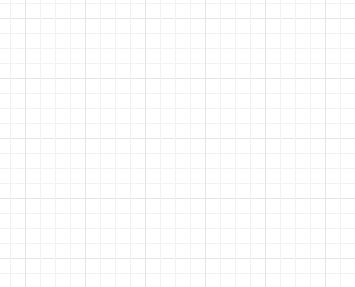RoI pooling layer需要两个输入：卷积特征图谱和候选区域坐标。但是在应用梯度下降时，该方法只能对特征图谱采用反向传播（BP）算法，而不能对候选区域坐标使用BP算法，为了克服这个缺点，在本文中，作者采用了双线性插值。
具体来说，就是对于任意的特征图谱$U（C×W’×H’）$和候选区域，作者要将其放缩成大小为$（C×X×Y）$的特征图谱$V$，放缩过程按照如下步骤进行：

1. 计算$V$到 $U$的反向投影坐标值，例如对于特征图谱$V$中的任意一点坐标$(x_{i,j}^V,y_{i,j}^V)$，投影到$U$中的坐标值为$x_{i,j}=x_{i,j}^V*\frac{W'}{X}，y_{i,j}=y_{i,j}^V*\frac{H'}{Y}$很容易看出，这里$x_{i,j}和y_{i,j}$的值均为浮点数，然而图像的像素坐标在计算机中必须为整数，所以这里坐标$(x_{i,j},y_{i,j})$对应的像素点是虚拟像素点，并不是$U$中实际存在的点。
2. 按照双线性插值法，得到$U$中$(x_{i,j}^U,y_{i,j}^U)$坐标点的像素值，该像素值就是$V$中对应点的像素值$V_{c,i,j}$，计算公式如下$V_{c,i,j}=\sum_{i'=1}^{W’}\sum_{j'=1}^{H'}U_{c,j',j'}k(i'-x_{i,j})k(j'-y_{i,j})，其中 ，k(d)=max(0,1-|d|)$
3. 利用上面的方法，计算$V$中所有像素点的坐标值，得到$C×X×Y$的特征图谱

对于上面的步骤可能理解起来不太直观，下面作者利用一个例子来帮助理解，作者假定源图谱U的大小为4×4，目的图谱V的大小为3×3，如下图所示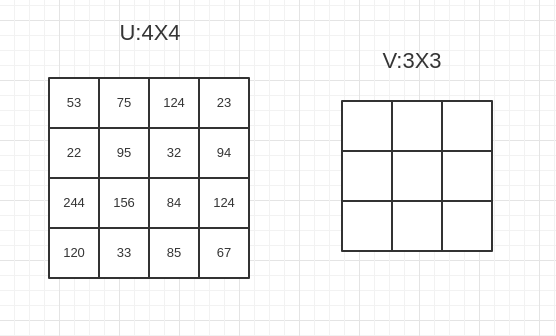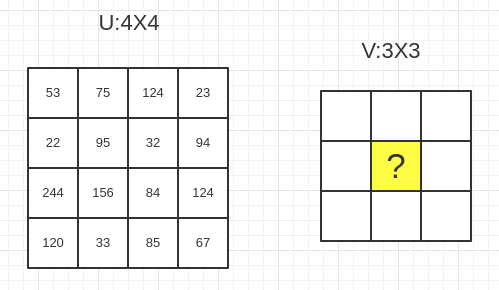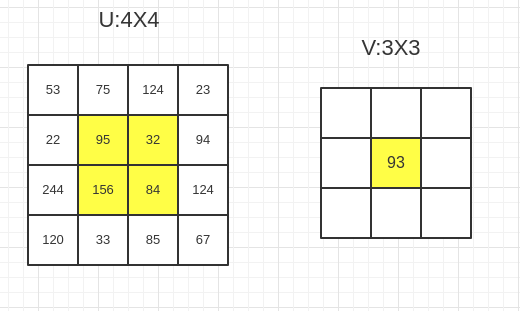### 识别网络（Recognition Network）

识别网络以一个全连接的神经网络，它接受的是来自定位层的候选区域的特征矩阵（定长）。将每个候选区域的特征拉伸成一个一维列向量，令其经过两层全连接层，每次都使用ReLU激活函数和Dropout优化原则。最终，对于每一个候选区域，都会生成一个长度为$D=4096$的一维向量。
将所有的正样本的存储起来，形成一个$B×D$形状的矩阵，将该矩阵传送到RNN语言模型中。

### RNN语言模型（RNN Language Model）

将图片的特征图谱输入到RNN语言模型当中，从而获得基于图片内容的自然语言序列。基本方法是将识别网络的输出结果进行编码（每一个候选区域到对应一个编码），记为$x_{-1}=CNN（I）$，然后将该区域对应的真实描述$s_1,…,s_T$也进行编码，记为$x_1,…x_T$，这里，$x_i$就是对应的$s_i$的向量编码。于是，作者就得到了长度为T+2的单词向量序列$x_{-1},x_0,x_1,…,x_T$，其中$x_{-1}$代表这候选区域的图像信息，$x_0$是特殊的开始标志，$x_1,…x_T$代表每一个单词的向量编码，将这T+2长度的向量序列feed到RNN中，训练出一个预测模型。

上式中，$x_{-1}$代表$CNN$生成的$D$维图像特征向量，并且它将作为整个$RNN$语言模型的初始输入，$S_t$代表RNN模型生成的一个个单词（word），其中$S_0$是一个特殊的开始标志，$p_{t+1}$代表第$t+1$个单词在整个单词表中的分布率，它是$p(S_{t+1}|I,S_0,…,S_t)$的简写形式，之后，选取$p_t$概率最大的元素作为句子中第$t$个单词的输出，如果概率最大的元素对应的是$END$标识符，则句子生成结束，迭代终止。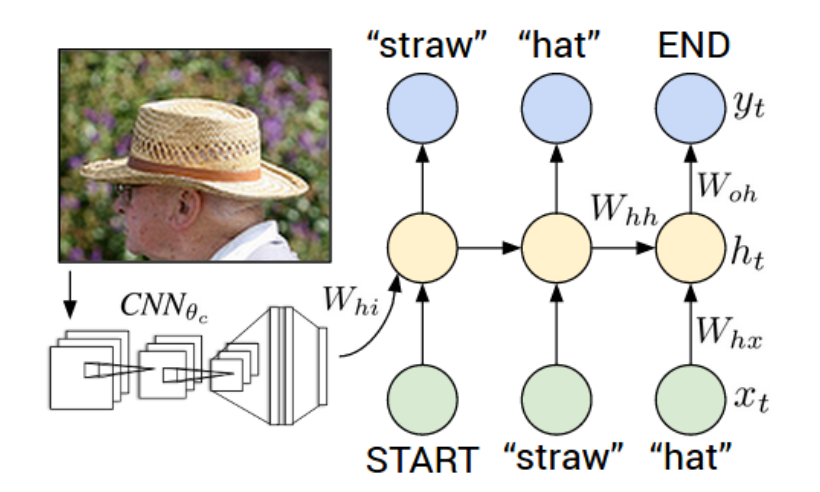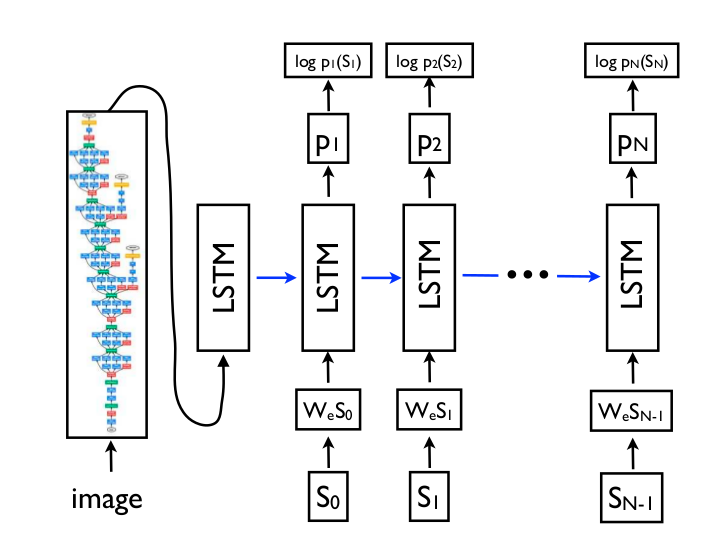Show and Tell: A Neural Image Caption Generator
https://arxiv.org/abs/1411.4555
Deep Visual-Semantic Alignments for Generating Image Descriptions
https://arxiv.org/abs/1412.2306

## 损失函数（Loss function）

这篇文章训练时的损失函数有五个，如下图所示，首先是lacalization layer定位层的边框位置回归和置信分数两处损失函数，前者使用smooth L1 loss，后者使用binary logistic loss。损失函数的数学定义可以参考Fast R-CNN和Faster R-CNN里面的损失函数。
接下来是Recognition Network的两处损失函数，该层和localization layer一样，也是边框位置和置信分数两个损失函数，最后是语言模型的损失函数，采用的取交叉熵（cross-entropy）损失函数。
作者利用bathch size和sequence length对所有的损失函数都进行了归一化。经过不断测试，作者发现将后续区域边框的初始权重设为0.1，将图片描述的置信权重设为1.0，是比较高效率的初始化设置。

: $l(w,b)=-\sum_{i=1}^{m}lnP(y_i|x_i;w,b)P(y=1|x)=\frac{e^{w^Tx+b}}{1+e^{w^Tx+b}}P(y=0|x)=\frac{1}{1+e^{w^Tx+b}}$这里，$w$为矩阵，$b$为向量，$x_i$是输入的图像区域的特征图谱，$y_i$为期望的真实输出（is or not object）

: $L_{loc}(t^u,v)=\sum_{i\in \{x,y,w,h\}}smooth_{L_1}(t_i^u-v_i)$

: $L(I,S)=-\frac {1}{T} \sum_{t=1}^{T}logp_t(x_t)$这里，$I$代表image，$S$代表sentence，$S_t$代表sentence中的第$t$个word，$p_t$代表第$t$个word的预测分布，因为要使损失函数最小，所以每次作者都选择概率最大的word。注意到这里的交叉熵损失函数比起完整的定义来说，缺少了真实分布P，这是因为在自然语言模型中，作者无法获取语句的真实分布，因此，需要用交叉熵的估计值进行代替。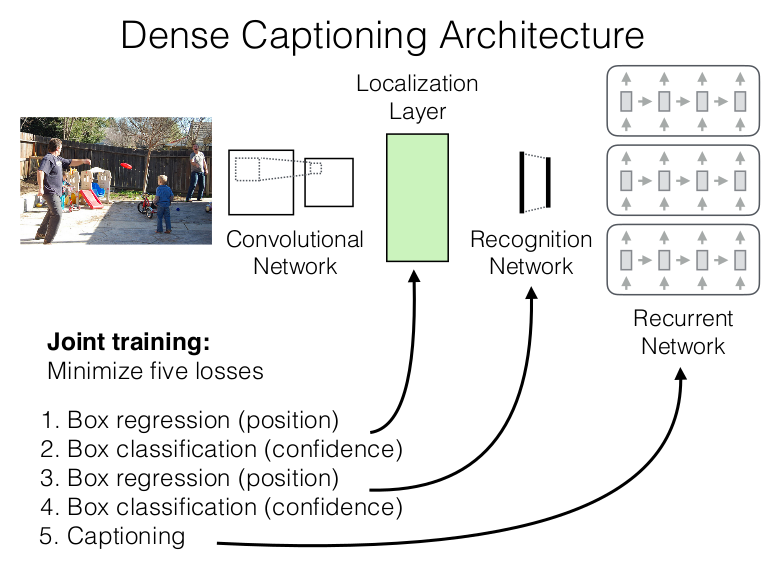## 训练和优化

作者用预训练ImageNet的权重来初始化CNN，其他权重的取值来源于标准差为0.01的高斯分布。CNN中权重的训练使用了momentum为0.9的SGD，其他的权重使用了Adam算法。学习速率分别为$\beta_1=0.9$和$\beta_2=0.99$。CNN的fune-tuning在迭代一轮后就开始了，但是不包含CNN中的前四个卷积层的微调。
训练时的batches由一张长边为720像素的图片构成（在该图片中选取B个候选区域组成mini-batch）。源码实现采用的是Torch7，在Titan X GPU上面一次mini batch的运行时间是300ms。训练模型直到收敛的运行时间总共花费了3天

# 实验